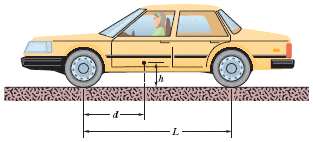# Static Equilibrium / Normal Forces / Braking Forces on tires

## Homework Statement

In the figure below, the driver of a car on a horizontal road makes an emergency stop by applying the brakes so that all four wheels lock and skid along the road. The coefficient of kinetic friction between tires and road is 0.36. The separation between the front and rear axles is L = 4.4 m, and the center of mass of the car is located at distance d = 1.8 m behind the front axle and distance h = 0.75 m above the road. The car weighs 11 kN. Find the magnitude of the following. (Hint: Although the car is not in translational equilibrium, it is in rotational equilibrium.)(a) The braking acceleration of the car.
(b) The normal force on each rear wheel.
(c) the normal force on each front wheel.
(d) the braking force on each rear wheel.
(e) the braking force on each front wheel.

$f_k = .36$
L = 4.4m
d = 1.8m
L-d = 2.6m
h = .75m
W = 11,000 N
m = 1,121.30 kg

## Homework Equations

$F_Friction = f_k * F_N F = ma \tau = {r_\bot}F \tau = rF_\bot$

## The Attempt at a Solution

a) $f_k * F_N = .36 * 11000 = 3960 N$ (Correct)
b)$\tau_{front} + \tau_{back} = \tau_{Total}$
I use the front wheel as the moment so \tau_front will be 0.
$0 + rF_{back}_\bot = 1.8*F_{N}_{Total}$
4.4F_back_\bot = 1.8(11000)
F_back_\bot = 4500[/itex]
So $F_N$ each wheel would be 2250 N, if it was correct (it isn't). Obviously no point in doing c) if I don't have the concept down.

for d) and e) I assume you do the same kind of conservation of torque, except you use braking force instead of normal force?

Thanks. Sorry about the broken itex, too pissed atm to fix it.

PeterO
Homework Helper

## Homework Statement

In the figure below, the driver of a car on a horizontal road makes an emergency stop by applying the brakes so that all four wheels lock and skid along the road. The coefficient of kinetic friction between tires and road is 0.36. The separation between the front and rear axles is L = 4.4 m, and the center of mass of the car is located at distance d = 1.8 m behind the front axle and distance h = 0.75 m above the road. The car weighs 11 kN. Find the magnitude of the following. (Hint: Although the car is not in translational equilibrium, it is in rotational equilibrium.)(a) The braking acceleration of the car.
(b) The normal force on each rear wheel.
(c) the normal force on each front wheel.
(d) the braking force on each rear wheel.
(e) the braking force on each front wheel.

$f_k = .36$
L = 4.4m
d = 1.8m
L-d = 2.6m
h = .75m
W = 11,000 N
m = 1,121.30 kg

## Homework Equations

$F_Friction = f_k * F_N F = ma \tau = {r_\bot}F \tau = rF_\bot$

## The Attempt at a Solution

a) $f_k * F_N = .36 * 11000 = 3960 N$ (Correct)
b)$\tau_{front} + \tau_{back} = \tau_{Total}$
I use the front wheel as the moment so \tau_front will be 0.
$0 + rF_{back}_\bot = 1.8*F_{N}_{Total}$
4.4F_back_\bot = 1.8(11000)
F_back_\bot = 4500[/itex]
So $F_N$ each wheel would be 2250 N, if it was correct (it isn't). Obviously no point in doing c) if I don't have the concept down.

for d) and e) I assume you do the same kind of conservation of torque, except you use braking force instead of normal force?

Thanks. Sorry about the broken itex, too pissed atm to fix it.

Just checking: was the Reaction force on the rear wheels given as 3825 N → 1922.5 each?

Last edited:
Yes, they're equally balanced on either side of the CoM.

PeterO
Homework Helper
Yes, they're equally balanced on either side of the CoM.

My real question referred to the quantity.

You had referred to your answer 4500 N [divided 2250 N each wheel] as being incorrect, ad you were not sure why it was incorrect.

I had tried a slightly different approach and got 3825 N , so divided equally between the back wheels.

I was querying the 3825 N [or was it 3845?] as the correct answer gven - as it did not seem appropriate to enter a lengthy analysis to teh wrong answer.

PeterO
Homework Helper
Yes, they're equally balanced on either side of the CoM.

Assuming my suggested answer was correct - I proceded as follows.

I took rotation around the centre of mass.

The Upward force on the front wheels is clockwise.

The upward force on the rear wheels, and the firction force - whether it be provided by the front or rear wheels - are both anticlockwise.

Although the equation derived above has two unknowns, we also know that the sum of the upward force on the front wheels and back wheel totals the weight of the vehicle, so simultaneous equation methods can be used to solve.

At first I only calculated the upward force on the rear wheels - but it is simple to calculate the force on the front, and then split the braking force into friction force on appropriate wheels.

Yes, your calculation for the reaction force on rear wheels was right. How did you get that? I think I understand how you found the rest, but that's not clear to me.

PeterO
Homework Helper
Yes, your calculation for the reaction force on rear wheels was right. How did you get that? I think I understand how you found the rest, but that's not clear to me.

Did my second post explain?